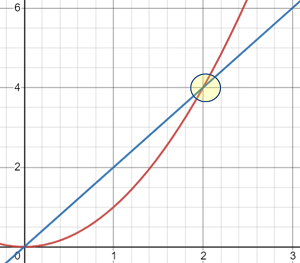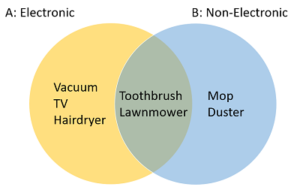# Intersection of Two Lines, Sets: Find by Hand, TI-89/Graph

Problem Solving >

Contents:

## What is an Intersection in math?

An intersection is the point where two or more things meet. In math, we’re usually interested in where graphs or sets meet.

•Intersection of two graphs (yellow highlight).The intersection of a graph is the point (x, y) where two functions cross each other on a graph.
•A∩B = Toothbrush, lawnmower.The intersection of two sets X and Y (X ∩ Y) is the set containing all elements of X that also belong to Y.
•In statistics, you might also want to find the intersection of a table (like the z-table) to find z-scores.

## Intersection of Two Lines

You may want to find the intersection of two lines for many reasons. Perhaps the most important one is that the intersection of two graphs is the solution to a system of equations; If you’re more of a visual learner it’s much easier than solving systems of equations algebraically.

Three ways to find the intersection of two lines (click to skip to that section):

## Find the Intersection of two lines on a TI-89The point where the two graphs cross each other (intersect) is the solution to the system.

## How to Use Intersection and Trace on the TI 89

Using a TI 89 to find the intersection is much faster than the hand method and is no harder than pressing a few buttons.

## Intersection Feature

Example problem: find the intersection of two functions:

• f(x) = x2 + 3x + 7
• f(x) = x2 + 5x + 9

Step 1: Press HOME.

Step 2: Press the diamond key and then F1 to enter into the y=editor. Next, press the CLEAR button if there are any values in the y1 slot and then press ENTER to go down to the input line.

Step 3: Enter the first function/equation. For this example, press x ^ 2 + 3 x + 7.

Step 4: Press ENTER to enter the function into the “y1 =” slot.

Step 5: Enter the second function. Press x ^ 2 + 5 x + 9.

Step 6: Press ENTER . This puts the second function into the “y2 =” slot.

Step 7: Press HOME.

Step 8: View the graph by pressing the diamond key and then F3 . You will see that the two graphs intersect. Note: If you don’t see a graph, press F2 and then press 6.

Step 9: Press F5 and then 5 to select “Intersection.”

Step 10: When you are asked “1st curve?” press ENTER.

Step 11: When you are asked “2nd curve?” press ENTER.

Step 12: For the lower bound, press the left arrow, moving the arrow to the left of the intersection. Then press ENTER.

Step 13: For the upper bound, arrow to the right of the intersection and press ENTER.

The TI-89 will give you an “x” value of -1 and a “y” value of 5. The intersection of these two graphs is (-1,5).
That’s it! You’re done!

## Trace Feature

The trace feature can come in handy to find your place on the graph.

Step 1: Press F3 for the Trace feature.

Step 2: Press the left arrow or the right arrow to trace along the graph. Change which graph you trace along by pressing the up or down arrows.

Step 3: To see a particular value for the function, press the desired value and then press ENTER. For example to see what y equals for an x-input of 4, press 4 and then press ENTER.

That’s it! You’re done!

## How to Find the Intersection of Two Lines Algebraically

Example problem: Find the intersection for the linear functions f(x) = 3x + 2 and f(x) = 2x -1.Step 1: Set the equations equal to each other.
3x + 2 = 2x – 1
Step 2: Solve for x to find the x-intersection.

• 3x + 2 = 2x – 1
• Subtract 2 from each side: 3x = 2x – 1 – 2
• Add 2x to each side: 3x – 2x = -1 – 2
• Simplify: x = -3

The x-intersection is -3.
Step 3: Use the value you found in Step 2 to find y. Take one of the original equations (we’ll use 3x + 2) and plug in the x-value:

• y = 3x + 2
• y = 3(-3) + 2 = -7

The intersection of two lines is (-3, -7).

## Intersection of Sets

The intersection of sets is the set of all elements the sets have in common.

One way to find the intersection of two sets is to write out all the elements and highlight those that appear in both sets.
For example: A = (1, 2, 3, 4) and B = (3, 4, 5, 6). The intersection of A and B is:
A∩B = (3, 4).

If there is no intersection at all, it’s called a null or empty set.

Another way to find the intersection of two sets is to draw a Venn diagram.

An Intersection is a collection of elements (like people, places, or things) that belong to two or more sets.A Venn diagram showing household objects that could be classified as either electronic or non-electronic. The intersection contains toothbrushes and lawnmowers, which belong to both sets.

The intersection is like a container with the elements as the contents. The container itself says nothing about the probability that an intersection of two (or more) events will happen. For that, you’ll need to calculate the joint probability.

If the events don’t have any outcomes in common, they are called pairwise disjoint or mutually exclusive events. The two terms are sometimes used to mean the same thing, but there is an important difference in set theory:

• Pairwise disjoint events have an intersection that is the empty set.
• Mutually exclusive events have a zero probability of happening at the same time.

## How to Draw an Intersection (A ∩ B)

Visually, items in an intersection appear in both categories at the same time.

Example question: Draw the intersection of the following black and white objects:

• Cats,
• Coal,
• Hair,
• Milk,
• Onyx,
• Shaving Cream,
• Snow,
• Tar.

Step 1: Place the items into categories (in this case, “black things” and “white” things):

• Black things: Cats, Coal, Hair, Tar, Onyx.
• White things: Cats, Hair, Snow, Milk, Shaving Cream.

Step 2: Draw two or three circles (i.e. a Venn diagram) according to how many categories you have. Make sure the circles overlap, because that’s where the intersection is.

Step 3: Write the items that the lists have in common (i.e. those that are in the intersection) in the space where the circles overlap.
For this example, that’s {cats, hair}.

Step 4: Fill in all of the other items to complete the Venn diagram.That’s it!